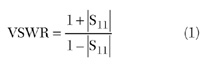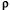Improving VSWR is typically an iterative trial-and-error exercise when utilizing either frequency or time domain information. For example, while circuit modifications which decrease time domain reflectometry (TDR) "bumps" (discontinuities) are generally a good thing, decreasing a specific TDR bump does not necessarily improve VSWR within a frequency band of interest; in fact, it can actually make it worse.By using information in both domains concurrently, however, one can determine the specific TDR discontinuities which contribute to specific VSWR lobes. This requires a vector network analyzer (VNA) because vector data is necessary to transform to a TDR display, and the phase information in the S11 data is necessary to find the correct time alignment of the TDR data.

Note that the terms VSWR and S11 will be used interchangeably throughout this article, but it is specifically S11 that contains the necessary phase information. VSWR and |S11| are just alternative representations of reflected energy; although scaled differently, both measured increase with increasing reflected energy.#### Relationship between TDR and S11

There are multiple versions of TDR measurements and transforms. On a VNA, the time domain measurements are not direct TDR measurements, but are transforms of the frequency domain reflection data. The most useful one here is the "low pass step transform." This is a menu choice on VNAs such as Agilent's performance network analyzer (PNA) and 8510 vector network analyzers, and is chosen because it clearly identifies capacitive, inductive and resistive discontinuities, as shown in Figure 1 . Note that the term "capacitive discontinuity" refers implicitly to a region of excess shunt capacitance, while "inductive discontinuity" refers implicitly to a region of excess series inductance.

Mathematically, the low pass step transform is the integral of the inverse Fourier transform of the windowed vector S11. This mathematical relationship is the key to relating the TDR discontinuities to the VSWR lobes.

Consider first the case of a TDR with a single small capacitive discontinuity at t = 0. This is a negative bump in the low pass step transform. If any other capacitive bumps are added at t = M, where M is an integer andis any arbritrary time interval, VSWR lobes are created at f = N/, where N is also an integer. This is because the phase at f = N/due to each capacitive bump is the same, so the energy addition is coherent and maximized. This is most easily understood since the delay between capacitive bumps is an integer number of cycles at f = N/. Similarly, if any inductive bumps (positive in the low pass step transform) are added at t = (M+0.5), they will also add coherently to the lobe at f = 1/and its odd harmonics; the combination of their inverted time amplitude (relative to the capacitive bumps) and their half-cycle delay result in S11 phase equal to that due to the capacitive bump, and therefore constructively adding.

A TDR measurement will reveal a variety of discontinuities of varying size, spacing and type (capacitive, inductive, or resistive). Nonetheless, the S11 lobes represent those frequencies where the energy is most constructive (or coherent), and the VSWR local minima are those frequencies where the energy is most cancelled. By properly specifying the TDR measurement, based on information from the S11 measurement, those TDR bumps which are coherent, as well as those which are canceling, can be positively identified.

#### Measurement Specification

Frequency domain measurements of VSWR or S11 typically show a series of lobes. As previously described, these are predominantly caused by phasing, due to the combination of size, type and delay of the set of time domain discontinuities.

According to Murphy's Law, one or more lobes are most likely to be placed in the frequency band where the design requires the best VSWR. Clearly, the tuning efforts should be focused on those circuit elements that are coherently contributing to the problem lobe(s). The measurement setup for the Agilent 8510 and PNA vector network analyzers is as follows:

1. Ensure a valid 1-port calibration has been performed on the VNA. (First, press the "SET FREQ (LOW PASS)" key in the transform menu, to ensure that both domains are calibrated.)

2. Set Channel 1 to "Low Pass Step Transform," and Channel 2 to S11. (For the PNA, use only one channel, and configure two "measurement traces" as specified above.)

3. Find f0, the frequency at the peak amplitude of the largest S11 lobe in the frequency band of interest.

4. Set "Electrical Delay" to 0, then find the phase of S11 at f0; denote this phase(in degrees).

5. Set "Electrical Delay" in both channels (or traces) to (90-)/(360f0). The S11 lobe phase should now read 90°.

6. Set "Channel 1 Span" to 10/f0, "Center" to 0.4Span, "Format" to "Real," "Ref Position" to 5 divs, "Ref Value" to 0 and "Scale" to 0.05 units/div. (For the PNA, the time trace "Center" and "Span" are set in the "Transform" dialog box.)This measurement setup provides a very powerful visual tool in focusing circuit modifications on those circuit discontinuities that directly (coherently) contribute to the problem VSWR lobe. Figure 2 shows an example of the TDR results for the case where the problem VSWR lobe is at 2.2388 GHz.

With this setup, all coherent discontinuities are positive (inductive) on the x-axis gridlines, or negative (capacitive) at the midpoints between the x-axis gridlines. Points at 25 or 75 percent within an x-axis division are orthogonal to the lobe energy and therefore of very low contribution; in fact, the orthogonal energy across the entire TDR must sum to zero at the lobe frequency since the transformed phase is exactly 90° (after electrical delay adjustment) for the low pass step transform.

Equally important, any capacitive bumps on gridlines are actually canceling energy at the lobe of interest; therefore, it would be a mistake to fix them. This is also true for any inductive bumps at the midpoints between the x-axis gridlines. The figure contains examples of coherent, orthogonal and canceling discontinuities.

These relationships can be easily verified using a small capacitive probe, and moving it along the electrical path. By observing the TDR and S11 displays concurrently, it is clear that the relationships of discontinuity location and type to S11 lobe magnitude follow the previously described rules.

Those familiar with the Fourier transform may wonder why the 90° condition is used for the time alignment rather than 0°. The reason is that the low pass step transform is the time integral of the low pass impulse response, which is the direct transform of the broadband S11. The time integral corresponds to a 90° shift in S11. The time integral also explains why the magnitude of successive VSWR lobes almost always increases with frequency; the time derivative in the converse definition generates a 2f multiplier in S11.

#### Calibrating the Displayed TDR Discontinuities

For the purpose of designing circuit modifications to correct or compensate identified TDR bumps of interest, it is important to know the magnitude of excess capacitance, inductance, or resistance causing the bump. Standard RF/microwave design tools can then be used to design correctly sized circuit features for discontinuity correction or compensation.

For resistance discontinuities, the resistance (or impedance) change iswhere= TDR amplitude immediately before the resistive discontinuityt+ = amplitude immediately after the resistive discontinuity

For small resistive discontinuities,R2Z0for< 0.1 (3)

For capacitive discontinuities, the low pass step transform in an infinite bandwidth system would be(t) = -u(t)e-2t/(C·Z0) (4)

For C = 0.1 pF and Z0 = 50, the time constant of this exponential pulse is 2.5 ps with a corresponding bandwidth of about 64 GHz. Therefore, an 18 GHz VNA will limit the bandwidth of the pulse, lowering the amplitude and spreading it. Furthermore, the S11 information is windowed prior to transformation to prevent ringing in the TDR, reducing the TDR bandwidth to about 7 GHz, depending on the window chosen in the transform setup. The displayed capacitive bump is the time convolution of the VNA transform impulse response with the ideal response of Equation 4.For small capacitive discontinuities (that is, < 0.2 pF), the peak amplitude of the measured capacitive bump is approximately proportional to the excess capacitance. With specific knowledge of the frequency domain window, this relationship can be derived analytically. Practically, however, it is simple to calibrate it using a stub of known capacitance. Figure 3 shows the analytical relationship specifically for an 18 GHz span when using the "NORMAL" transform window in the 8510 VNA, which, in the PNA, corresponds to a Kaiserwindow factor of six. In this case, the excess capacitance is approximately

CKc|pk| for |pk| < 0.1 (5)

where the constant of proportionality for capacitance is

Kc = 1.9 pF

Inductive discontinuities behave the same as capacitive discontinuities, but with positive amplitude. Once the proportionality constant for capacitance has been determined as in Equation 5, the proportionality constant for inductance can be calculated as

KL = Z02Kc (6)

And the relationship for excess inductance is similarly

LKL|pk| for |pk| < 0.1 (7)

where, in this case, KL = 4.75 nH

#### Correction and Compensation of the Circuit Discontinuities

There are two ways to improve VSWR. Either correct the specific circuit discontinuities diagnosed in the described method, or, with knowledge of the magnitude of the excess capacitance, inductance or resistance, design correctly sized compensating circuit features.

Clearly, correcting the discontinuities is preferred whenever possible. For example:

• transmission line transitions from one PCB layer to another
• transmission lines passing close to grounded PCB features
• transmission line bends with non-optimum chamfers
• transmission line launches in or out of components, including the effect of component terminations and the PCB pads.

"Compensation" is required when it is not possible to get at the discontinuity itself. A common example of this is the GaAs FET switch, which has capacitive, inductive and resistive discontinuities within the component itself. In reality, all lumped components have discontinuities that are within the part and cannot be fully corrected at the component ports. How well they can be compensated depends on how close the compensation can be located to the discontinuity and on the compensation topology; this is particularly true for broadband circuits.

#### Conclusion

A deterministic method of VSWR optimization has been presented. The method requires a broadband VNA with time domain transform capability for concurrent measurements of S11 and low pass step transform. The method steps are:

1. Identify the largest VSWR lobe in the frequency band of interest; use S11 phase data to find the correct electrical delay required to align the low pass step transform data.

2. Configure the Low Pass Step Transform span and center time to place coherent inductive discontinuities on gridlines, and coherent capacitive discontinuities at grid midpoints.

3. Identify the problem discontinuities. Wherever possible, correct the discontinuity or apply compensation. Calibration of the discontinuity magnitude allows the design of correctly sized compensating features.

4. Repeat the procedure for any other problem VSWR lobes in the frequency band of interest, in order of decreasing lobe magnitude.

This method eliminates the vast majority of trial-and-error iteration required by methods relying solely or serially on time domain or frequency domain measurement. It is the concurrent methodology and measurement capability that enables positive determination of those circuit discontinuities that coherently contribute to any given VSWR lobe.

Tim Hillstrom received his BSEE degree from the University of Portland and his MSEE degree from the University of Washington. He has been with Agilent (formally Hewlett-Packard) for 20 years, working on circuit and subsystem design of spectrum analyzers, vector signal analyzers and wireless base/mobile station test sets. He is currently working on the design of next-generation wireless mobile test sets.